Courses

# Calculation of Tender or Quotation - Overheads, Cost Accounting B Com Notes | EduRev

## B Com : Calculation of Tender or Quotation - Overheads, Cost Accounting B Com Notes | EduRev

The document Calculation of Tender or Quotation - Overheads, Cost Accounting B Com Notes | EduRev is a part of the B Com Course Cost Accounting.
All you need of B Com at this link: B Com

How to Calculate Tender Price or Quotations?
Very often a manufacturer or producer is asked to submit a tender or cost-estimate for the supply of the product in future. The price quoted for future production is called Quotation Price or Tender Price. This price is ascertained on the basis of previous cost sheet or production account. In ascertaining expected cost in the future, the items of previous elements of cost are considered with due regard to expected changes in the future. Estimated cost is increased by desired profit to ascertain tender price or quotation.

Tenders of Similar Type Commodity:
When cost of same type and quality of commodity is to be calculated, normally cost per unit of each element of cost will be taken up. If there is any expected change in these elements, the change will be adjusted accordingly. Following examples will explain the technique.

How to Calculate Tender Price or Quotations?
Illustration l: On I Slh August, 2005 the Standard Cycle Co was required to quote for a eon tract for the supply of 500 bicycles From the following details, prepare a statement showing the price to he quoted to give the same percentage of net profit on turnover as was realised during the si\ months to 311th lune 2005.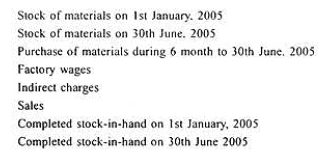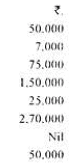The number of bicycles manufactured during the six months was 2.000 including those sold and those in stock at the end of the period. The bicycles to be quoted for are to be of uniform size and quality and similar to those manufactured during the six months to 30th June 2005. As from 1st August, the cost of factory labour has increased by 10 per cent and that of materials by 15 per cent.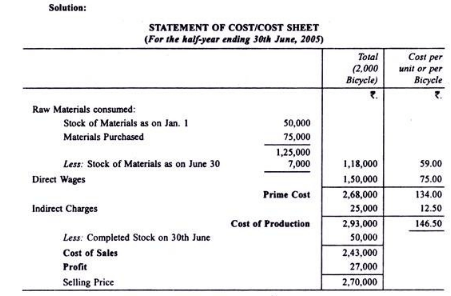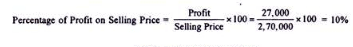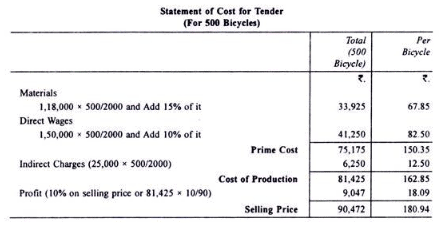Tenders for Different Product:
When the tender or quotation price is to be ascertained for a different product, the cost of direct material, direct labour and other direct expenses will be estimated. The total of these will be prime cost Works overheads, office overheads and selling overheads will be added there to on the basis of absorption rates. Normally works overhead is charged or absorbed on the basis of percentage of works overhead on wages. Thus percentage is calculated on the basis of past records.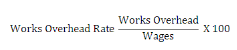It may be alternatively charged on the basis of Machine Hour Rate or Labour Hour Rate. Office overheads may be absorbed on the basis of percentage of office overheads on works cost or works overheads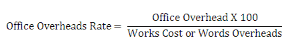The total of Prime Cost plus absorbed works overheads and office overheads will be Cost of Production. Selling overheads, if any, may be added there to on the basis of its absorption rate. It may be a percentage on cost of production or sales or at selling overheads per unit. By adding desired profit. Tender Price or Quotation Price will be known.

Following examples will explain the technique: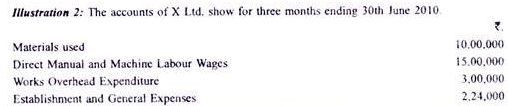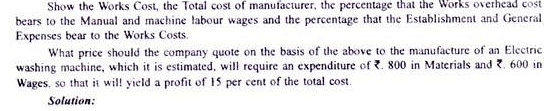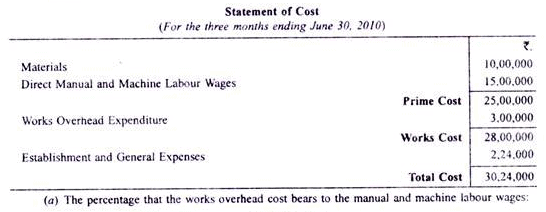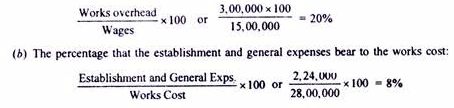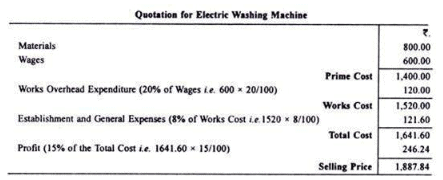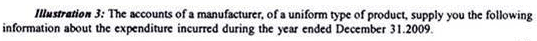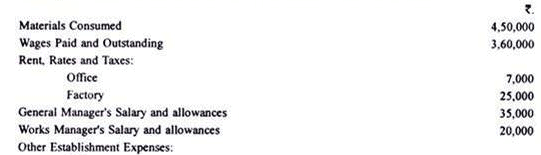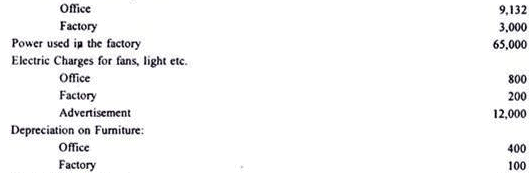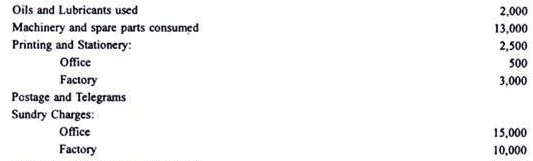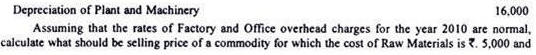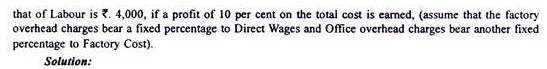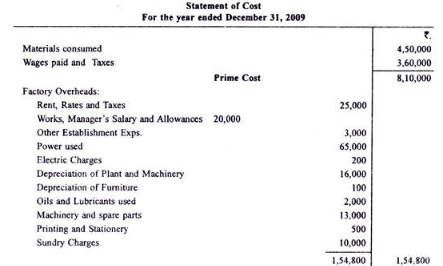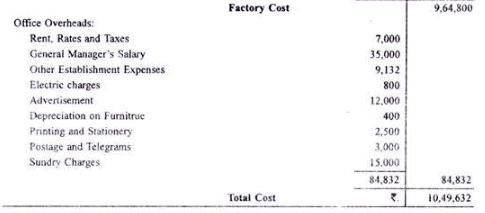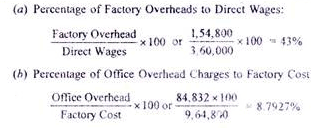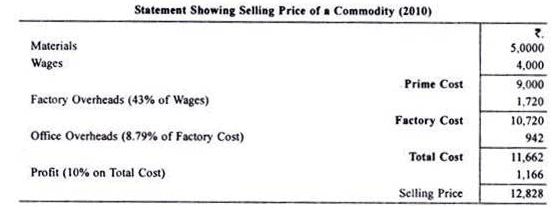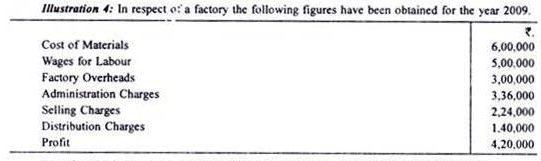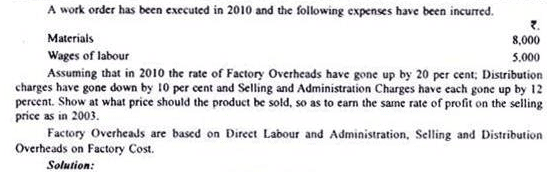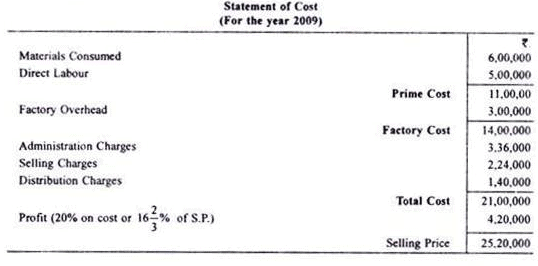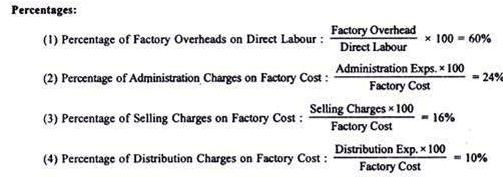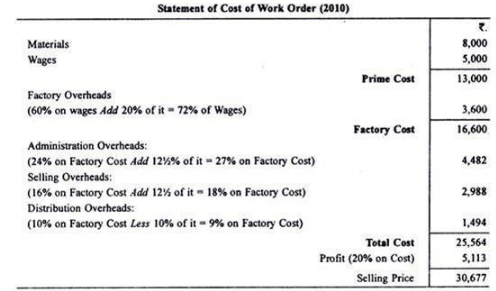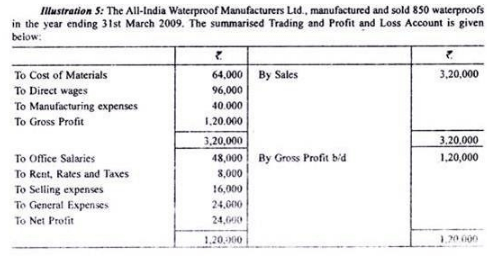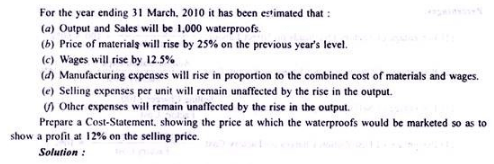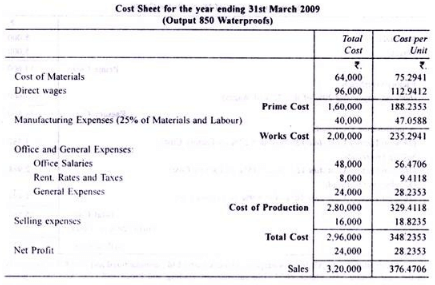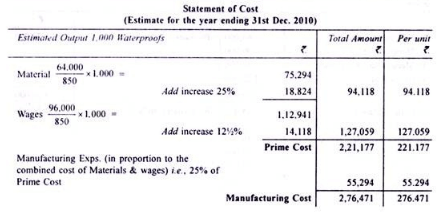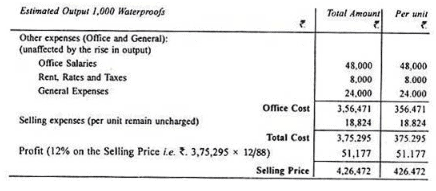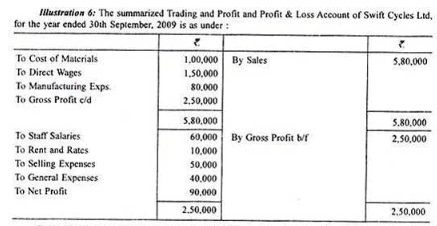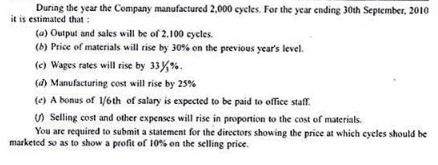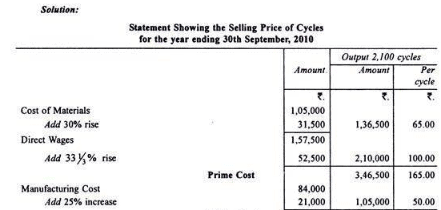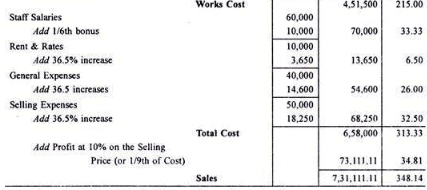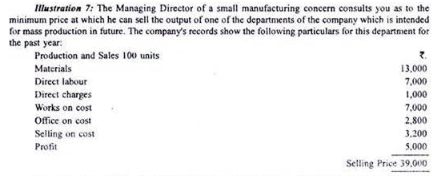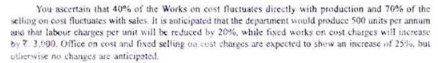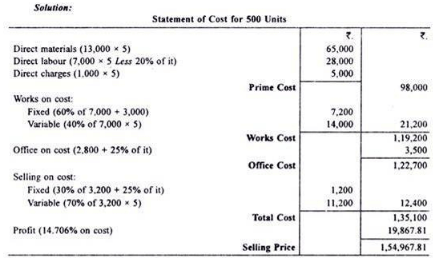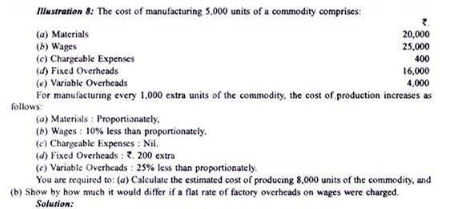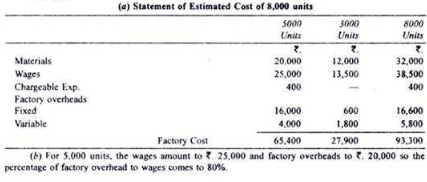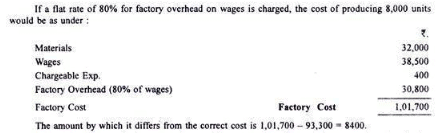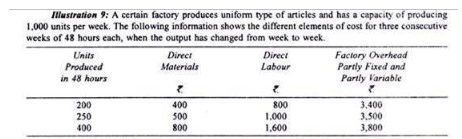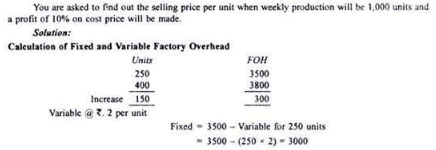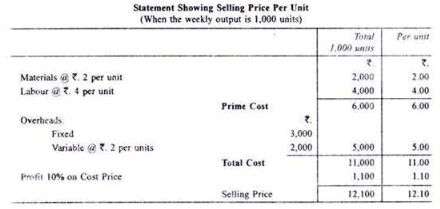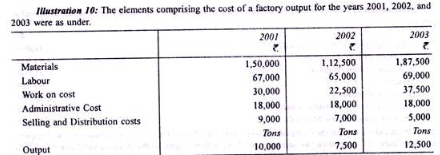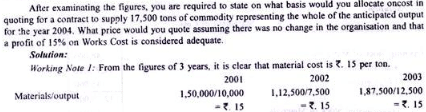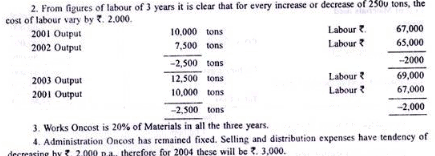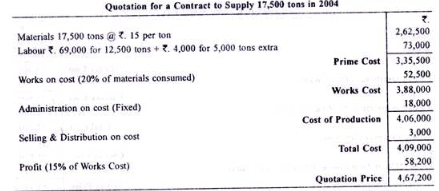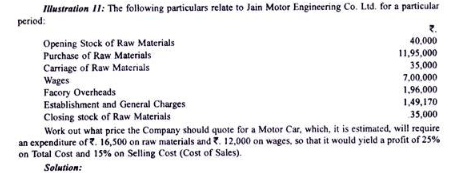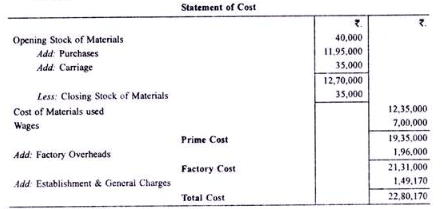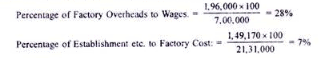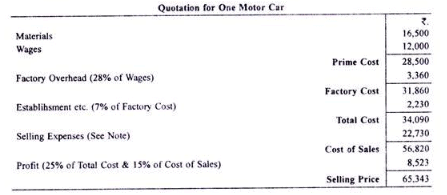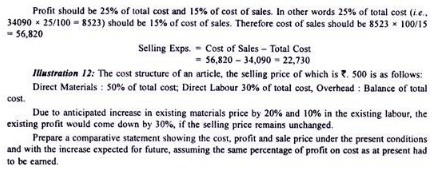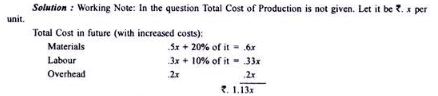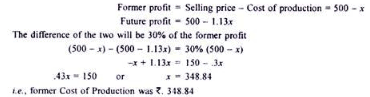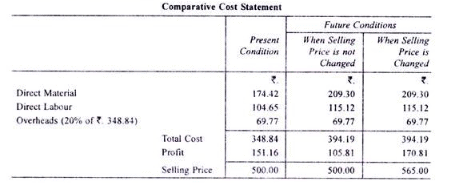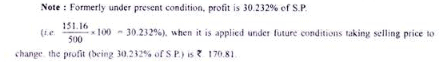Difference Between Quotation and Tender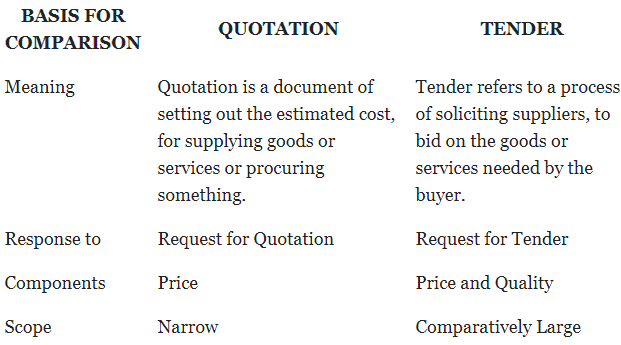Calculation of Profit:
(i) Calculation of Profit at Cost Price:
For this, the following formula is adopted –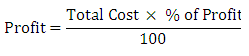This can be explained clearly with the help of following example
Total Cost Rs. 18,000
Percentage of Profit 10%
Solution:
1. Profit at Cost Price: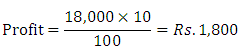2. Profit at Selling Price when Cost Price is Given: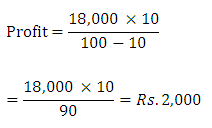Requirements for the Preparation of Tender or Quotation:
For the preparation of a tender or quotation, a manufacturer must have detailed information regarding the cost of raw materials, wages and the different overheads and the profit of the preceding period. It is only on the basis of the cost and profit data of the identical product produced in the immediate past period, a producer can make an estimate of the cost of the product after making adjustments for the likely changes in the price levels of various components of production and submit the tender or quotation for the goods.

Steps to be Taken for the Preparation of Tender or Quotation:
The preparation of tender or quotation can be considered under two heads i.e.:
(1) If the quantity of the identical product produced in the past and the quantity of the product for which tender is prepared are stated in the problem, and
(2) If the quantity of the identical product produced in the past period and the quantity of the product for which the tender is prepared are not stated in the problem.

I. Calculation of Tender Price on the Basis of Previous Cost per Unit (When Quantity is Given):
In this case, for the preparation of the tender the following steps will have to be taken:
First Step – Preparation of the Cost Sheet for the Past Period:

As the tender has to be prepared on the basis of the cost of identical product produced in the past period, and as the output of the past period is given, first, we will have to prepare a cost sheet with total cost column and cost per unit column for the past period with the help of the costing data relating to the past period.
Second Step – Calculation of Percentages of Overheads, and the Percentage of Profit on Sales of the Past Period:
After the preparation of the cost sheet for the past period, we have to calculate the percentage of factory overheads on direct wages, and the percentage of office and selling overheads on works cost of the past period, if we are specifically instructed that the factory overheads and office and selling overheads for the tender are to be taken at the same percentages as in the past period.
However, if such a specific instruction is not given, we need not calculate the percentage of factory overheads on direct wages, and the percentages of office and selling overheads on works cost of the past period, as the overheads of tender can be calculated on the basis of the overheads per unit of the past period.

Calculation of Percentages:
The above percentages can be calculated as follows:
(a) Percentages of Factory Overheads on Direct Wages: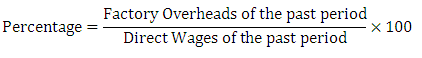(b) Percentage of Office and Selling Overheads on Works Cost: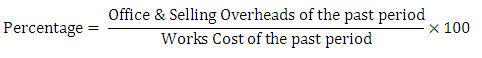(c) Percentage of Profit on Sales of the Past Period:
Beside the overhead percentages, we have to calculate the percentage of profit on sales of the past period, if the problem does not state the percentage of profit to be taken into account for the preparation of the tender or if the problem specifically states that the tender is to be made at the same percentage of profit on sales in the past period.
However, if the problem specifically states the specific percentage of profit to be taken into account for the preparation of the tender, we need not calculate the percentage of profit on sales of the past period, as the tender is to be made at the given percentage of profit and the percentage of profit on sales of the past period is of no relevance for the preparation of the tender.
If the percentage of profit on sales of the past period is required for the preparation of the tender, it can be calculated as follows: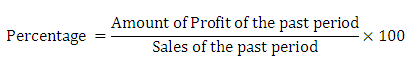In this context, it may be noted that the percentage of profit on sales of the past period can be calculated only if the amount of profit of the past period is known. The amount of profit of the past period can be ascertained by preparing a statement of profit for the past period.
Another point to be noted in this context is that the percentage of profit on sales to be taken into account for the preparation of the tender cannot be taken as it is, as we know only the cost price, and not the selling price of the product for which the tender is to be made. So, what we actually require for direct application to the tender is the percentage of profit on cost.
The percentage of profit on cost to be applied to the tender directly can be calculated from the percentage of profit on sales required to be taken into account for the preparation of tender, it can be found out as follows: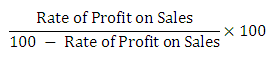Third Step – Preparation of the Statement of Tender:
After the calculation of the overhead percentages and the percentage of profit on cost to be taken into account for the preparation of tender, we have to prepare the statement of tender with cost per unit column and total cost column.
While preparing the statement of tender, the various expenses required to be taken into account for the preparation of tender can be found out either on the basis of the specific cost figures given for the tender or on the basis of the unit cost of past period and the quantity of product for which tender is given subject to necessary adjustments for current changes in the rates of the various elements of cost, or on the basis of the overheads percentage of the past period.
After ascertaining the various expenses on relevant basis or bases, the various elements of cost have to be added. The resulting figure is the total cost. With the total cost, we have to add the amount of profit required to be taken into account for the tender on the basis of the percentage of profit given or on the basis of profit on sales of the past period, converted into the percentage of profit on cost. The resulting figure is the amount of tender or the quotation price.

II. Calculation of Tender Price on the Basis of the Percentage of Previous Expenses (When Quantity is not Given):
In this case, for the preparation of the tender, the following steps have to be taken:
First Step – Preparation of the Statement of Cost of the Past Period:
As the tender has to be prepared on the basis of the cost of identical product produced in the past period, and as the output of the past period is not given, first, we have to prepare only a statement of cost with just one amount column for the past period with the help of the costing data relating to the past period.
Second Step – Calculation of the Percentages of Overheads and the Percentage of Profit on Sales of the Past Period:
After the preparation of the statement of cost for the past period, we have to calculate the percentage of factory overheads on direct wages, and the percentage of office and selling overheads on works cost of the past period; as the overheads required to be taken into account for the tender have to be calculated on the basis of the relevant overhead percentages of the past period.
As explained in the previous case, the percentage of factory overheads on direct wages and the percentage of office and selling overheads on works cost can be calculated as follows:
(a) Percentage of Factory Overheads on Direct Wages: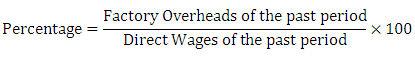(b) Percentage of Office and Selling Overheads on Works Cost: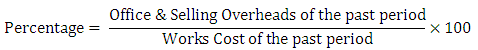(c) Percentage of Profit on Sale Price, if Sales Price is given: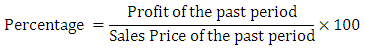(d) Amount of Profit to be Calculated on Selling Price at a Certain Percentage, when Sales Price is Not Given: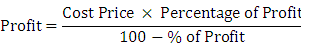Third Step – Preparation of the Statement of Tender:
After the calculation of the overhead percentages and the percentage of profit on sales price or cost price required to be taken into account for the tender, we have to prepare a statement offender with the help of the costing data relating to tender given in the problem and with the help of the overhead percentages and the percentage of profit on cost.

(III) Estimation by Taking into Consideration the Fixed and Variable Expenses:
Elements of cost under the nature of cost, there are three kinds of costs on the basis of nature:

1. Fixed Cost:
Fixed cost is that which is unaffected due to increase or decrease in the volume of output. For example – Factory rent, Manager’s salary, etc. Thus, in absence of proper direction, fixed cost remains same at each production level.
2. Variable Cost:
Variable cost is that which is directly related with the output. In other words, in case of increasing production it increases and it decreases when the production is decreased.
3. Semi-Variable Cost:
Those expenses the part of which is fixed and the next part of which is variable, are called semi-variable costs. They are not directly concerned with the production. They vary less or more than proportionately.
It should be kept in mind while solving tender price questions, directions given in this regard should not be avoided.

Offer running on EduRev: Apply code STAYHOME200 to get INR 200 off on our premium plan EduRev Infinity!

## Cost Accounting

131 videos|139 docs

,

,

,

,

,

,

,

,

,

,

,

,

,

,

,

,

,

,

,

,

,

,

,

,

;